# PIXILAB Wiki

Tasks can be used to build many useful functions, ranging form simple sequences of commands or complex logic involving conditions, loops and variables. You program tasks with a mix of drag-and-drop and JavaScript expression syntax. A basic command sequence is programmed by dragging do and wait statements into a list and selecting values from dropdown menus.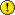For an overview of Tasks, please refer to the corresponding chapter in the Blocks manual. This article contains more in-depth information on the expressions that can be used in tasks.

### Parameters

Many commands require additional values, called parameters. Such values are typed into fields in the statements. An example of such a value is the duration of a wait, which is entered as a number, specified in seconds.In many cases, you'll just type a number into such a parameter field, such as the number 1 in the illustration above. However, all such parameter fields can accept an expression, which is a formula that can be calculated, or evaluated, to a value of the desired type. For instance, you can type the following into the wait parameter field:

`1 + 1.3`

This simple formula evaluates to 2.3 seconds, which is then used by the wait statement. Of course, a simple formula such as 1 + 1.3 has no advantage over simply typing 2.3 into the field directly. Since all parts that make up that expression are literal numbers, the result of the expression will always be the same (it's a constant expression). The true power of expressions can only be unlocked with the help of variables.

### Trigger Condition

In addition to the parameters used in statements, expressions are also used in the Trigger Condition field found at the top of each Task.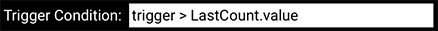This expression can be used to further qualify under what conditions the task should run. Thus, in order for a task to start, the following must be true:

1. It's Trigger must fire (such as "Property Change", "Time of Day" or "Server Startup").
2. Any Trigger Condition must evaluate to true.

While the Trigger is the mere occurrence of the specified event (such as the time being 8:00 in the morning), the Trigger Condition can apply arbitrarily complex conditions on top of this event in order to decide whether to actually start the task. For instance, in the case of a time-of-day trigger, you can specify that it should only start the task if it's Saturday and a certain Block is playing on a particular Spot.

A Trigger Condition often uses the predefined variable named trigger, as in the example shown above. This variable is further described below under "Predefined Variables". You can, however, use any system property in the condition.

## JavaScript Expressions

Expressions in Blocks use JavaScript syntax. There are numerous excellent sources of information on this subject, such as this one:

Here we'll only provide a brief overview of the syntax, enough to get you started with programming of Tasks in Blocks.

### Data Types and Literals

The wait example above used a simple expression of the form 1 + 1.3, which obviously results in the value 2.3. This is a mathematical formula, involving two numbers and the add operator. This is a familiar type of expression for most people. However, in JavaScript, number is only one type of data.

Another important data type is a string of characters, often called just a string. This data type is used to handle textual values, such as the name of a cue in WATCHOUT, as shown in the illustration.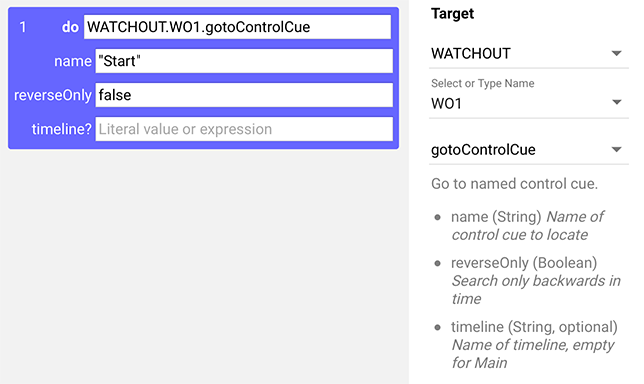Note that the types of parameters are shown in the bottom right corner, here as name (String) followed by a brief description of the parameter. To specify a literal string, enclose it in quotes, as shown above. Blocks will put the quotes there for you automatically for mandatory parameters, to remind you. But in some cases (such as the timeline parameter, which is optional), you need to remember to do so yourself. Looking at the type of parameter expected will guide you.

Yet another type often encountered is boolean. A boolean value represents something that is either false or true, so it has only two possible outcomes. An example is the reverseOnly parameter shown in the illustration above.

So, to summarize, these are the data types you may encounter when working with Tasks:

• Number, such as 3.14 or -88656. Note that you must use a decimal point to separate any fractional part, not a comma.
• String, such as "Start" or 'HDMI'. You can use either double-quotes or single quotes around a string literal.
• Boolean, such as false and true. These words must not be surrounded by quotes (or they would be considered Strings, which isn't the same thing).

The examples given above all use literals. A literal represents only its own, constant value. A formula involving only literal values isn't particularly useful, except as an example, since it will always evaluate to the same result. Thus, to create more dynamic behaviors, you often mix literal and variable values, as discussed below.

#### Truthy Expressions

JavaScript has a fairly lax syntax in many areas, such as for Boolean expressions. While you may prefer to be explicit when providing boolean values, JavaScript will accept pretty much anything here, attempting to interpret it as true or false. This is often referred to as truthy or falsey in JavaScript documentation. Examples of truthy expressions include:

• true (literally true)
• -5 (non-zero number)
• 3+9.5 (non-zero number)
• "Arne" (non-empty string)

Examples of falsey expressions include:

• false (literary false)
• 0 (zero)
• 3+2-5 (zero)
• "" (empty string)

While this behavior may be used as a convenient shortcut, it's often better to write an explicitly boolean expression, as it more clearly expresses your intention.

### Optional Parameters

Task statements may need multiple parameters. The gotoControlCue statement accepts up to three parameters:

1. The name of the cue to go to (String).
2. Whether to search forward and then backwards, or backwards in time only (Boolean).
3. Which timeline to control (String).Of these three parameters, only the first two are required. An Optional parameter is indicated by a question mark following its name, and is also marked as optional in the description in the right hand corner. In this case, leaving out the timeline name will target the Main Timeline, as stated in the description.

If there are multiple optional parameters, you may only leave out trailing optional parameters. Thus, if there are four parameters, of which the last two are optional, you may omit parameter 3 and 4, or only parameter 4. You can't leave out only 3, while specifying parameter 4.

### Operators

An expression typically consists of a mix of literal values (constants), operators and variables. When writing a numeric expression (one that is expected to yield a numeric result), you can use all common operators, such as:

• + and - for addition and subtraction.
• * and / for multiplication and division.
• ( and ) for grouping sub-expressions to express order of evaluation.

There are many more operators that can be used with numbers, but those are the most common ones. You also have access to the JavaScript standard objects Math and Number. For instance, if you want to make sure you get a whole number, rather than a number with fractions, as a result of an expression, you can use the Math.round function, like this:

`Math.round(trigger / 7)`

#### Relational Operators

Another type of operators allow you to compare things, such as numbers. As they evaluate the relation of two values to each other, they are often called relational operators. They all result in a boolean value. Examples of relational operators include:

• < for "left is less than right".
• > for "left is greater than right".
• == for "left is equal to right".
• != for "left is not equal to right".

For example, in the trigger condition of a task triggered by time of day, you can write:

`trigger.getDay() != 0`

This uses the fact that the trigger variable (more on that below under "Predefined Variables") of a task triggered by time of day is a Date object, which provides a getDay() method, returning the number of the weekday represented, where 0 is Sunday. Thus this task will run any day except Sunday. That is, the day number returned by the getDay() method is not equal to 0. Learn more about the date object here:

#### Boolean Operators

Sometimes you want to combine multiple boolean sub-expressions. For instance, you may want to trigger a task only on weekdays, but not weekends. Unfortunately, the getDay() method of the Date object (discussed above) yields 0 or Sunday and 6 for Saturday, so you can't easily select for weekdays with a single comparison. Instead you must check for these days individually, and then combine the result, like this:

`trigger.getDay() != 0 && trigger.getDay() != 6`

Which reads "day is not equal to 0 and day is not equal to 6", which does exactly what we want.

Here are some common boolean operators:

• && for and.
• || for or.
• ! for not.

The && and || operators take two values (one on each side of the operator). Thus, if you want to run a task only on weekends, you could write a condition like this:

`trigger.getDay() == 0 || trigger.getDay() == 6`

Which reads "day is equal to 0 or day is equal to 6".

The not (exclamation point) operators shown above is known as a unary operator as it takes a single value (also called operand), which follows after the operator. The not operator inverts the boolean value of its single operand. Thus, if the value that follows it is falsey, the result will be true and vice versa.

For instance, assume you want to trigger a task only if an contact closure input is not activated, then you could write a condition like this

`IO.motion1.value != true`

Here, motion1 is the name of the contact closure input. Since this is a simple switch input, it is known to have a boolean value (either it's ON or it is OFF). So if the input is ON (i.e., true), the above expression will evaluate to false, as we're saying "input's value is not equal to true".

But you could just as well have written the above as

`!IO.motion1.value`

As the input is already known to be a boolean value, all we need to do here is to "invert" it. Thus, the above expression will be true if the signal is OFF (i.e., false). Whether you use the shorthand unary not operator or the explicit comparison to true is mostly a matter of taste.

#### String Operators

The most commonly used operation with strings is to concatenate them. This is done using the plus operator, like this:

`"PIXI" + "LAB"`

This results in a single string containing the text PIXILAB. Again, doing this with only literal operands makes little sense, as you could just as well type the resulting string directly as "PIXILAB". However, if one of the values come from a variable, it makes more sense. For example, you can log the value of an input like this (assuming here it's given the IO alias motion1):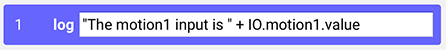#### Operator Precedence

Just as in math, some operators take precedence over others. For example, multiplication is done before addition. The same applies to relation and boolean operators. Here's a table showing the full list of JavaScript operators, arranged from highest to lowest precedence:

Whenever in doubt, you can always use parenthesis to explicitly specify the order ot evaluation. For example to add two numbers together first, and then multiply the intermediate result with another number, you can write:

`(5 + 3) * 9`

## Variables

The true power of expressions comes from the use of variables. A variable essentially names a "mailbox" in which a value is stored. When you reference the name of that "mailbox", what you get is the value in it. In Blocks, you can use pretty much any state in the entire system as such a value source. For instance, if you have a contact closure input connected through a Modbus box, the state of that input can referenced.

As an example, to trigger a task only if a certain input signal is present, simply refer to the value of that input in the trigger condition:

`IO.motion1.value`

The same applies to any value supplied as a parameter to a statement. For example, to keep flashing a light (here controlled through a Modbus output) for as long as an input signal is present, do this: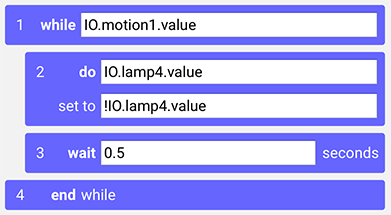This example shows a number of useful patterns:

• It uses a while statement to repeat a group of statements for as long as its expression is true.
• It uses a do statement to set the state of an output.
• It uses the unary not operator (the initial exclamation point, see above) to toggle the state of the output by using the inverse of its current state as the parameter.

### Predefined Variables

• trigger contains the value of whatever triggered the task.
• now contains the current time and date, as a JavaScript Date object.

The actual value of the trigger variable depends on what kind of trigger the task uses:

• If the triggering mode is “Time of Day”; it's a JavaScript Date object.
• For “Property Change” it's a number, boolean, string or other object indicating the property’s new state.
• For "Server Startup", the value of trigger is undefined.

If no trigger is assigned to the task, the value of trigger is undefined, and should not be used. Note that trigger will contain the current value of the tasks trigger even if the task was started by other means, such as manually clicking the play arrow next to the task's name, or by setting the task's running property to true.

### Realm Variables

You can add externally visible variables to a Realm. When doing so, you specify the data type used by that variable (number, boolean or string). Such a variable can be set frmo the outside, for example using a button on a panel, or by another task (using a set realm variable statement).

The value of such a realm variable can be used as a trigger, just like any other system property. You can also directly reference the value of a realm variable in any task within the same realm using the name of the variable followed by a period and the work value. Assuming you have a realm variable named Brightness, you can use its value in an expression like this: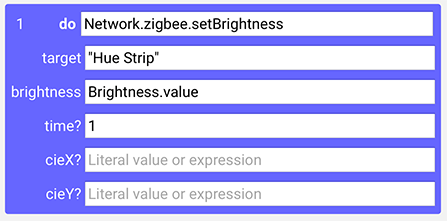When referencing the value of a realm variable, always remember to type the trailing .value part.

### Local Variables

A local variable is often useful to hold intermediate results, or other values you want to use multiple times in a task. For example, to create a simple lighting chaser effect, you can use a while loop with the sequence of statements needed to control the lights, interspersed with wait statements to control the speed of the effect. If you're unsure of what speed will work best, you can assign the value to a local variable called speed, and then use that variable in the wait statements: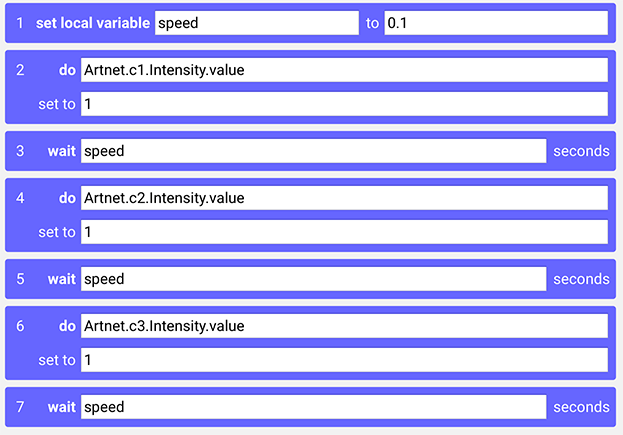Now, to try a different speed of this chaser effect, simply change the value of the speed variable, rather than changing the value of each individual wait statement, since they all refer to the same variable.The value of a local variable is referenced simply by using its name.

### Property References

As seen in some of the examples above, you can set the value of any property in your Blocks system using a do statement. You can pick the target of the do statement using the dropdown menus to the right. Alternatively, if you know the full path to the desired property, you can type this into the do statement's target field.

Some properties are read only. An example of such a read only property is the connected state of a Display Spot. There's nothing you can do from within Blocks to force a Spot to connect. Thus, this property can only be read, not set. Read only properties are shown in italics on dropdown menus. They can not be used as the target property of a do statement. They can, however, be used in expressions and task triggers.

The value of a system property can also be directly used in expressions. Since such an expression typically consists of more than just a single property reference, you need to enter the full path to the property manually here.

#### Obtaining Property References

It can sometimes be hard to figure out or remember the full path to a property you want to use in an expression. In this case, you can copy the property reference from somewhere else, such as a task trigger. Simply make an empty "dummy task", set it to trigger on Property Change, pick the desired property using the dropdown menus, then copy the entire property path from the Custom Path field,

## Using Target Expressions

Not only can you use expressions in the parameter fields of task statements. You can also use expressions in the target field of a do statement. This follows the JavaScript equivalence of dot notation and bracket notation. For example, the target in the first do statement under "Local Variables" above is specified as

`Artnet.c1.Intensity.value`

An alternative form with the same meaning is

`Artnet["c1"].Intensity.value`

Here the dot notation is replaced with the "array index"-style bracket notation, with a string expression inside the brackets. While this form, using a string literal, has no advantage over the simpler dot notation, it can be extended further by using an expression inside the brackets.

For example, assume you have a numeric realm variable named channel that specifies which lighting channel to control, as a number. As shown in the example under "Local Variables" above, there are a number of lighting channels, named c1, c2 and c3. You can then use an expression combining the leading "c" in the channel name with the numeric value of the realm variable like this:

`Artnet["c" + channel.value].Intensity.value`

The expression inside the brackets performa string concatenation, so that if the channel realm variable contains 2, the resulting string will be "c2", thus controlling the channel with that name.